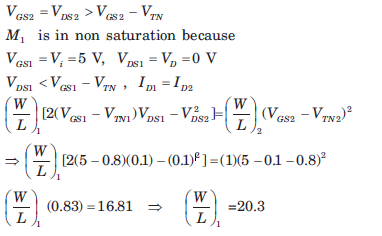Courses

# Basic FET Circuits - MCQ Test

## 15 Questions MCQ Test GATE ECE (Electronics) 2022 Mock Test Series | Basic FET Circuits - MCQ Test

Description
This mock test of Basic FET Circuits - MCQ Test for Electronics and Communication Engineering (ECE) helps you for every Electronics and Communication Engineering (ECE) entrance exam. This contains 15 Multiple Choice Questions for Electronics and Communication Engineering (ECE) Basic FET Circuits - MCQ Test (mcq) to study with solutions a complete question bank. The solved questions answers in this Basic FET Circuits - MCQ Test quiz give you a good mix of easy questions and tough questions. Electronics and Communication Engineering (ECE) students definitely take this Basic FET Circuits - MCQ Test exercise for a better result in the exam. You can find other Basic FET Circuits - MCQ Test extra questions, long questions & short questions for Electronics and Communication Engineering (ECE) on EduRev as well by searching above.
QUESTION: 1

### In the circuit shown in fig. the transistor parameters are as follows: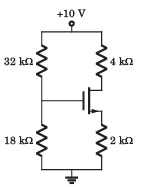Threshold voltage VTN = 2V Conduction parameter Kn = 0.5mA/V2 Que: VGS = ?

Solution: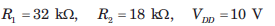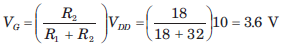Assume that transistor in saturation region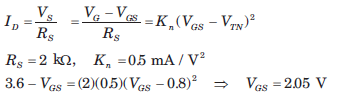QUESTION: 2

### In the circuit shown in fig. the transistor parameters are as follows:Threshold voltage VTN = 2V Conduction parameter Kn = 0.5mA/V2 Que: ID =?

Solution: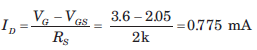QUESTION: 3

### In the circuit shown in fig. the transistor parameters are as follows:Threshold voltage VTN = 2V Conduction parameter Kn = 0.5mA/V2 Que: VDS = ?

Solution: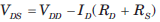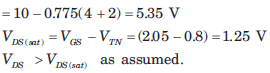QUESTION: 4

In the circuit shown in fig. the transistor parameter are as follows: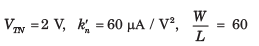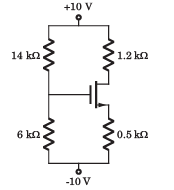Que:

VGS =?

Solution: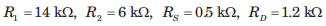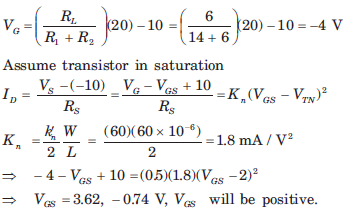QUESTION: 5

In the circuit shown in fig. the transistor parameter are as follows:Que: ID = ?

Solution: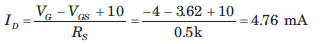QUESTION: 6

The parameter of the transistor in fig.  are VTN = 1.2 V, Kn = 0.5mA / V2 and λ = 0. The voltage VDS is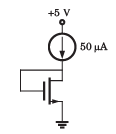Solution: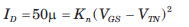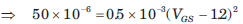VGS = 1.52 V, VGS = VDS

QUESTION: 7

The parameter of the transistor in fig. are VTN = 0.6  V  and Kn = 0.2 mA / V2 . The voltage VS is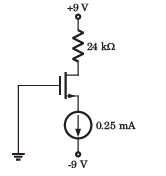Solution: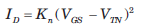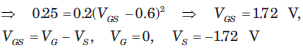QUESTION: 8

In the circuit of fig. the transistor parameters are VTN = 1.7 V and Kn = 0.4mA / V2.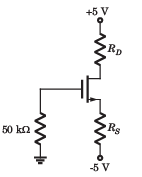If ID = 0.8 mA and VD = 1 V, then value of resistor RS and RD are respectively

Solution: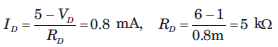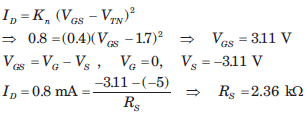QUESTION: 9

In the circuit of fig. the PMOS transistor has parameter VTP = 1.5 V, kp' =  25 μA/V2 , L = 4 μm and λ = 0.If ID = 0.1 mA and VSD = 2.5 V, then value of W will be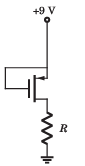Solution: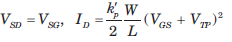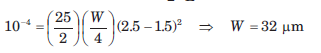QUESTION: 10

The PMOS transistor in fig. has parameters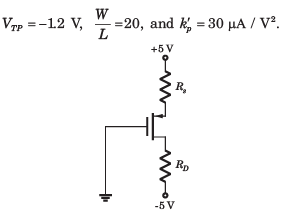Solution: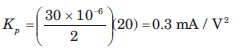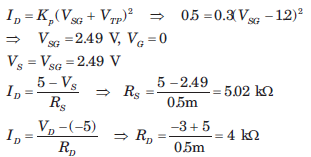QUESTION: 11

The parameters for the transistor in circuit of fig. are VTN = 2 V and Kn = 0.2mA / V2. The power dissipated in the transistor is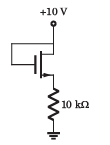Solution:

Assume transistor in saturation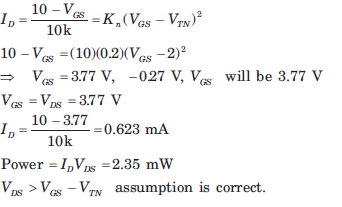QUESTION: 12

Consider the circuit shown in fig.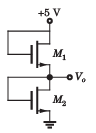The both transistor have parameter as follows

VTN = 0.8V, kn' = 30 μA/V2

Que: If the width-to-length ratios of M1 and M2 are

(W/L)1 = (W/L)2 = 40

The output Vo is

Solution:

For both transistor VDS = VGS ,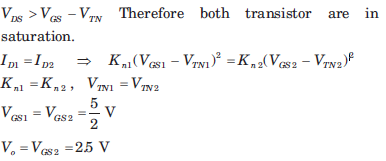QUESTION: 13

Consider the circuit shown in fig.The both transistor have parameter as follows

VTN = 0.8V, kn' = 30 μA/V2

Que: If the ratio is (W/L)1 = 40 and (W/L)2 = 15, then v0 is

Solution: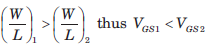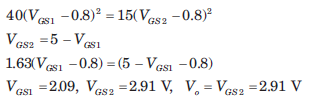QUESTION: 14

In the circuit of fig. the transistor parameters are VTN =1 V and kn' = 36 μA/ V2 .If ID = 0.5 mA, V1 = 5 V and V= 2 V then the width to-length ratio required in each transistor is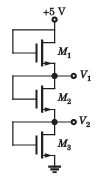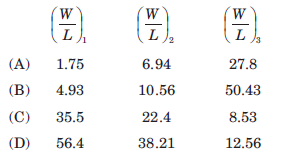Solution:

Each transistor is biased in saturation because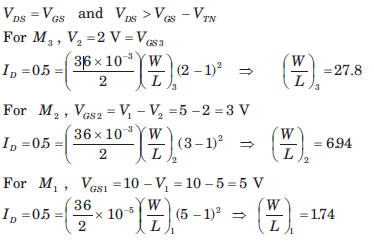QUESTION: 15

The transistors in the circuit of fig. have parameter  VTN = 0.8V, kn' = 40 μA/V2 and λ = 0 .The width-to-length ratio of M2 is (W/L)2 = 1. If Vo = 0.10V when vi = 5 V, then (W/L)1 for M1 is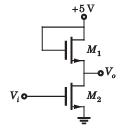Solution:

M2 is in saturation because